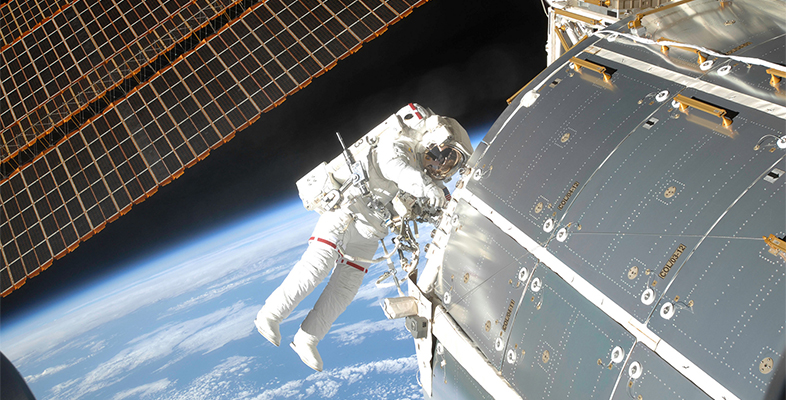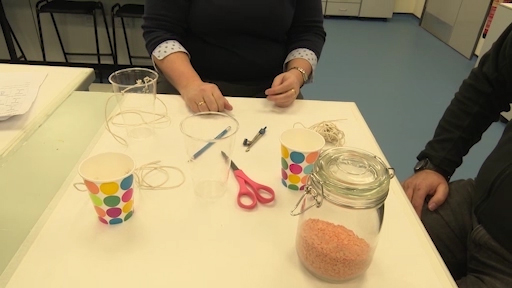Science, Maths & Technology

### Become an OU studentMicrogravity: living on the International Space Station

Start this free course now. Just create an account and sign in. Enrol and complete the course for a free statement of participation or digital badge if available.

# 4.1 Practical experiment 1Video 5 Demonstration of Practical Experiment.
Interactive feature not available in single page view (see it in standard view).

Practical experiment 1: Modelling how the ISS stays in motion around the Earth with the cup-of-rice experiment

To do this experiment, you need to be in a place with lots of space, ideally in a garden or a field. You will need a plastic cup, a piece of string and some rice. Cut two holes in the plastic cup opposite each other, thread the string through and tie it off. Half-fill the cup with rice. Taking care to avoid hitting anyone, swing this briskly around in a vertical circle.

To keep the cup moving, you need to exert a force (in the string, called tension). To increase the force on the cup, you need to increase the speed. If you put more rice into the cup, the mass has increased, so it will move slower. These are all factors which will change the motion of the cup. These variables are included in Equation 3 where F = force (N), m = mass (kg), v = velocity (speed) and r = radius (m).

Equation label: Equation 3

If the mass m increases, the force F increases (when r and v are constant).

If v increases, F increases (when r and m are constant).

However, if r increases, Fdecreases (when v and m are constant).

If m = 5 kg, and you swing it on a string where r = 1 m and v = 4 m/s, then, using Equation 3, you have a resultant force with a numerical value, as shown in Equation 4.

Equation label: Equation 4

Note that, while the forces are balanced in the rice cup experiment, the rice itself is in freefall while in motion, so that it doesn’t fall out of the cup. You know from Equation 3 that the units for force in Equation 4 should be N. This is the same as kg m/s2.

In terms of the orbit of the ISS, obviously there is no string connecting it to Earth! There must be some other kind of force acting on it. The gravitational pull of Earth is the force F acting here. However, if you then add the effects of friction from the atmosphere, the ISS slows down. It is no longer able to maintain the same gravitational orbit and you would have to either increase v or push it out to a higher orbit.